Courses

# Test: Problems On HCF And LCM- 2

## 10 Questions MCQ Test Quantitative Aptitude for Banking Preparation | Test: Problems On HCF And LCM- 2

Description
This mock test of Test: Problems On HCF And LCM- 2 for Quant helps you for every Quant entrance exam. This contains 10 Multiple Choice Questions for Quant Test: Problems On HCF And LCM- 2 (mcq) to study with solutions a complete question bank. The solved questions answers in this Test: Problems On HCF And LCM- 2 quiz give you a good mix of easy questions and tough questions. Quant students definitely take this Test: Problems On HCF And LCM- 2 exercise for a better result in the exam. You can find other Test: Problems On HCF And LCM- 2 extra questions, long questions & short questions for Quant on EduRev as well by searching above.
QUESTION: 1

### Find the lowest common multiple of 24, 36 and 40.

Solution: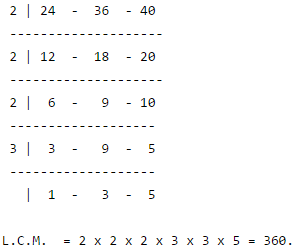QUESTION: 2

### The least number which should be added to 2497 so that the sum is exactly divisible by 5, 6, 4 and 3 is:

Solution: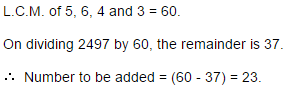QUESTION: 3

###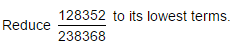Solution: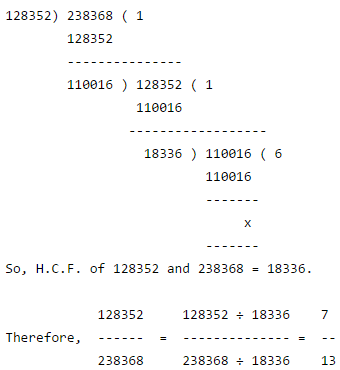QUESTION: 4

The least number which when divided by 5, 6 , 7 and 8 leaves a remainder 3, but when divided by 9 leaves no remainder, is:

Solution: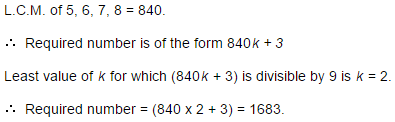QUESTION: 5

A, B and C start at the same time in the same direction to run around a circular stadium. A completes a round in 252 seconds, B in 308 seconds and c in 198 seconds, all starting at the same point. After what time will they again at the starting point ?

Solution:

L.C.M. of 252, 308 and 198 = 2772.

So, A, B and C will again meet at the starting point in 2772 sec. i.e., 46 min. 12 sec.

QUESTION: 6

The H.C.F. of two numbers is 11 and their L.C.M. is 7700. If one of the numbers is 275, then the other is:

Solution: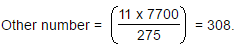QUESTION: 7

What will be the least number which when doubled will be exactly divisible by 12, 18, 21 and 30 ?

Solution: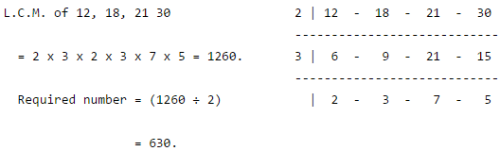QUESTION: 8

The ratio of two numbers is 3 : 4 and their H.C.F. is 4. Their L.C.M. is:

Solution:

Let the numbers be 3x and 4x. Then, their H.C.F. = x. So, x = 4.

So, the numbers 12 and 16.

L.C.M. of 12 and 16 = 48.

QUESTION: 9

The smallest number which when diminished by 7, is divisible 12, 16, 18, 21 and 28 is:

Solution:

Required number = (L.C.M. of 12,16, 18, 21, 28) + 7

= 1008 + 7

= 1015

QUESTION: 10

252 can be expressed as a product of primes as:

Solution:

Clearly, 252 = 2 x 2 x 3 x 3 x 7.